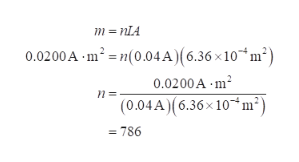# The orientation of small satellites is often controlled usingtorque from current - carrying coils in Earth’s magnetic field.Suppose a multiturn coil has a cross - sectional area of 6.36 =10-4 m2, dissipates 0.200 W of electrical power from a 5.00 - Vpower supply, and provides a magnetic moment of magnitude0.020 0 A · m2. (a) Find the coil current I. (b) Calculatethe number of turns in the coil. (c) Calculate the maximummagnitude of torque if Earth’s magnetic field has magnitude3.75 x 10-5 T at the satellite’s location.

Question
1 views

The orientation of small satellites is often controlled using
torque from current - carrying coils in Earth’s magnetic field.
Suppose a multiturn coil has a cross - sectional area of 6.36 =
10-4 m2, dissipates 0.200 W of electrical power from a 5.00 - V
power supply, and provides a magnetic moment of magnitude
0.020 0 A · m2. (a) Find the coil current I. (b) Calculate
the number of turns in the coil. (c) Calculate the maximum
magnitude of torque if Earth’s magnetic field has magnitude
3.75 x 10-5 T at the satellite’s location.

check_circle

Step 1

a)

Current is,

Step 2

Step 3

b)

Magnetic moment and number of ...help_outlineImage Transcriptionclosem = NIA 0.0200A m? = n(0.04A)(6.36 x10*m²) 0.0200A - m? (0.04A)(6.36× 10*m²) = 786 fullscreen

### Want to see the full answer?

See Solution

#### Want to see this answer and more?

Solutions are written by subject experts who are available 24/7. Questions are typically answered within 1 hour.*

See Solution
*Response times may vary by subject and question.
Tagged in

### Magnetic Effects of Current